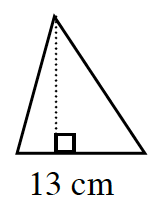### Home > ACC6 > Chapter 8 Unit 8 > Lesson CC1: 8.3.3 > Problem8-118

8-118.

If the area of this triangle is $100.75\text{ cm}^2$, what is the height?

$\text{Area of a triangle} =\frac{1}{2} (\text{base})(\text{height})$

Substitute all the values that are known.
$\frac{1}{2}(13)h=100.75$

Now solve for h.